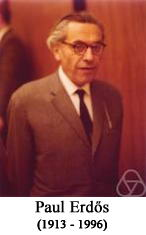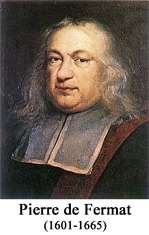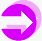Mixed Sums of Primes and Other Terms

visits since Feb. 2, 2009This webpage is maintained by Zhi-Wei Sun Please send your comments to zwsun@nju.edu.cnI. Mixed Sums of Primes and Polygonal Numbers

Conjecture on Sums of Primes and Triangular Numbers (Zhi-Wei Sun, 2008). (i) Each natural number n ≠ 216 can be written in the form p+Tx, where p is a prime or zero, and Tx=x(x+1)/2 is a triangular number with x nonnegative. (ii) Any odd integer greater than 3 can be written in the form p+x(x+1), where p is an odd prime and x is a positive integer.

Remark. Asymptotically the n-th prime is about n*log(n), so the conjecture looks harder than the famous Goldbach conjecture. Parts (i) and (ii) have been verified up to 1012 by T. D. Noe and D. S. McNeil respectively. Sun would like to offer 1000 US dollars for the first positive solution or \$200 for the first explicit counterexample.

General Conjecture on Sums of Primes and Triangular Numbers (Zhi-Wei Sun, 2008). Let a and b be any nonnegative integers, and let r be an odd integer. Then all sufficiently large integers can be written in the form 2ap+Tx, where p is either zero or a prime congruent to r mod 2b, and x is an integer. Also, all sufficiently large odd numbers can be written in the form p+x(x+1), where p is a prime congruent to r mod 2b, and x is an integer.

Examples for the General Conjecture on Sums of Primes and Triangular Numbers:
(1) (Zhi-Wei Sun, 2008) Any integer n>88956 can be written in the form p+Tx, where p is either zero or a prime congruent to 1 mod 4, and x is a positive integer.
(2) (Zhi-Wei Sun, 2008) Each integer n>90441 can be written in the form p+Tx, where p is either zero or a prime congruent to 3 mod 4, and x is a positive integer.
(3) (Zhi-Wei Sun, 2008) Except for 30 multiples of three (the largest of which is 49755), odd integers larger than one can be written in the form p+x(x+1), where p is a prime congruent to 1 mod 4, and x is an integer.
(4) (Zhi-Wei Sun, 2008) Except for 15 multiples of three (the largest of which is 5397), odd numbers greater than one can be written in the form p+x(x+1), where p is a prime congruent to 3 mod 4, and x is an integer.
(5) For a=0,1,2,... let f(a) denote the largest integer not in the form 2ap+Tx, where p is zero or a prime, and x is an integer. In 2008 Zhi-Wei Sun conjectured that f(0)=216, f(1)=43473 and f(2)=849591. In 2009 D. S. McNeil suggested that f(3)=7151445.

Remark. Jing Ma has verified the above (1)-(5) up to 1011.

Conjecture on Sums of Primes and Squares (Zhi-Wei Sun, 2009). If a positive integer a is not a square, then all sufficiently large integers relatively prime to a can be written in the form p+ax2, where p is a prime and x is an integer. In particular, any integer greater than one and relatively prime to 6 can be expressed as p+6x2 with p a prime and x an integer.

Remark. In 1753 Goldbach asked whether any odd integer n>1 is the sum of a prime and twice a square. In 1856 M. A. Stern and his students found counterexamples 5777 and 5993.

Conjecture on Sums of Primes and Polygonal Numbers (Zhi-Wei Sun, 2009). If a positive integer m>4 is not congruent to 2 mod 8, then all sufficiently large odd numbers can be written as the sum of a prime and twice an m-gonal number pm(n)=(m-2)n(n-1)/2+n (n=0,1,2,...). In particular, any odd integer n>1 other than 135, 345, 539 can be represented by p+2p5(x) = p+3x2-x with p a prime and x a nonnegative integer.

Remark. Let m>2 be an integer. In 1638 Fermat asserted that any natural number can be expressed as the sum of m m-gonal numbers; this was finally proved by Cauchy in 1813. In 2009 Zhi-Wei Sun conjectured that every natural number n can be written as the sum of an (m+1)-gonal number, an (m+2)-gonal number, an (m+3)-gonal number and a number among 0,...,m-3; in the case m=3 this has been verfied for n up to 3*107 by Qing-Hu Hou.

Related References
1. Zhi-Wei Sun, A new conjecture: n=p+x(x+1)/2, 2008.
2. Zhi-Wei Sun, A message to Number Theory List, 2008.
3. Zhi-Wei Sun, A general conjecture on sums of primes and triangular numbers, 2008.
4. Zhi-Wei Sun, Odd numbers in the form p+x(x+1), 2008.
5. Zhi-Wei Sun, Mixed sums of primes and triangular numbers, Journal of Combinatorics and Number Theory 1(2009), no.1, 65-76.
6. Zhi-Wei Sun, Offer prizes for solutions to my main conjectures involving primes, 2009.
7. Zhi-Wei Sun, Various new conjectures involving polygonal numbers and primes, 2009.
8. Zhi-Wei Sun, A challenging conjecture on sums of polygonal numbers, 2009.
9. Zhi-Wei Sun, A curious conjecture and a mysterious sequence, 2009.
10. Zhi-Wei Sun, On universal sums of polygonal numbers, 2009.
11. Jing Ma, Verifying SUN Zhi-Wei's conjectures on sums of primes and triangular numbers, Master Thesis (Anhui Normal Univ., China), 2009.
12. Z. W. Sun, Mixed sums of squares and triangular numbers, Acta Arith. 127(2007), no.2, 103-113.
13. B. K. Oh and Z. W. Sun, Mixed sums of squares and triangular numbers (III), J. Number Theory 129(2009), no.4, 964-969.
14. Y. Wang, Goldbach Conjecture, World Sci., Singapore, 1984.
15. Sequences A132399, A154752, A137996, A137997, A144590, A117054, A160324, A160325, A160326, A165141 in N. J. A. Sloane's OEIS (On-Line Encyclopedia of Integer Sequences).

II. Mixed Sums of Primes and Recurrences

The well-known Fibonacci numbers are given by
F0=0, F1=1, and Fn+1 = Fn + Fn-1 (n=1,2,3,...).
Here is the list of the initial 18 positive Fibonacci numbers:
1, 1, 2, 3, 5, 8, 13, 21, 34, 55, 89, 144, 233, 377, 610, 987, 1597, 2584.
The companion of the Fibonacci sequence is the sequence of Lucas numbers given by
L0=2, L1=1, and Ln+1 = Ln + Ln-1 (n=1,2,3,...).
It is easy to see that Ln = Fn+1 + Fn-1 for n=1,2,3,....

Conjecture on Sums of Primes and Fibonacci Numbers (i) (Weak Version) [Zhi-Wei Sun, Dec. 23, 2008 and March 19, 2009] Each integer n>4 can be expressed as the sum of an odd prime and two or three positive Fibonacci numbers.
(ii) (Strong Version) [Zhi-Wei Sun, Dec. 26, 2008] Each integer n>5 can be expressed as the sum of an odd prime, a positive Fibonacci numbers and twice a positive Fibonacci number. Also, any integer n>4 can be rewritten as the sum of an odd prime, a positive Fibonacci number and a Lucas number.

Remark. Since Fibonacci numbers and Lucas numbers grow exponentially, they are much more sparse than prime numbers. Thus the conjecture seems much more difficult than the Goldbach conjecture. It has been verified up to 1012 by D. S. McNeil (Univ. of London). Sun would like to offer \$5000 for the first positive solution or \$250 for the first explicit counterexample to the above conjecture or the conjecture (an earlier version) that any integer n>4 can be expressed as the sum of an odd prime and two positive Fibonacci numbers.

Values of the Representation Function r(n) for n=p+Fs+2Ft with s,t>1 (n=1,...,100000)

Values of the Representation Function r(n) for n=p+Fs+Lt (s>1, and Fs or Lt is odd) (n=1,...,100000)

Values of the Representation Function r(n) for n=p+Fs+Ft with s,t>1 and Fs or Ft odd (n=1,...,100000)

Values of the Representation Function r(n) for n=p+Fs+Ft/2 with s,t>1 (n=1,...,100000)

The Pell numbers are defined by
P0=0, P1=1, and Pn+1 = 2Pn + Pn-1 (n=1,2,3,...).
Here is the list of the initial 18 positive Pell numbers:
1, 2, 5, 12, 29, 70, 169, 408, 985, 2378, 5741, 13860, 33461, 80782, 195025, 470832, 1136689, 2744210.

Conjecture on Sums of Primes and Pell Numbers (Zhi-Wei Sun, Jan. 10, 2009). Any integer n>5 can be written as the sum of an odd prime, a positve Pell number and twice a positive Pell number.

Remark. This has been verified up to 5*1013 by D. S. McNeil. Using a variant of B. Poonen's heuristic arguments, on March 10, 2009 Sun predicted that there should be infinitely many positive integers congruent to 120 modulo 210 not of the form p+Ps+2*Pt with p an odd prime and s,t positive integers.

List of n ≤ 100000 in the Form Ps+2*Pt (s,t=1,2,3,...)

Values of the Representation Function r(n) for n=p+Ps+2Pt with s,t>0 (n=1,...,100000)

The Catalan numbers Cn=(2n)!/(n!(n+1)!) (n=0,1,2,...) play important roles in combinatorics. Asymptotically Cn4n / (n3/2π1/2). It is well known that
C0=1 and Cn+1 = C0Cn + C1Cn-1 + … + CnC0 for n=0,1,2,....
Here is the list of the initial 15 Catalan numbers:
1, 1, 2, 5, 14, 42, 132, 429, 1430, 4862, 16796, 58786, 208012, 742900, 2674440.

Motivated by the conjecture on sums of primes and Fibonacci numbers due to Sun, Qing-Hu Hou and Jiang Zeng made the following conjecture during their visit to Nanjing Univ.

Conjecture (Qing-Hu Hou and Jiang Zeng, Jan. 9, 2009). Every integer n>4 can be expressed as the sum of an odd prime, a positve Fibonacci number and a Catalan number.

Remark. Note that the generating function of the Cataln numbers is not rational. The conjecture has been verified up to 3*1013 by D. S. McNeil. Hou and Zeng would like to offer \$1000 for the first positive solution or \$200 for the first explicit counterexample.

Values of the Representation Function r(n) for n=p+Fs+Ct with s>1 and t>0 (n=1,...,100000)

The following conjecture is similar to the Hou-Zeng conjecture.

Conjecture (Zhi-Wei Sun, Jan. 16, 2009). Any integer n>4 can be written as the sum of an odd prime, a Lucas number and a Catalan number.

Remark. This has been verified up to 1013 by D. S. McNeil.

Values of the Representation Function r(n) for n=p+Ls+Ct with t>0 (n=1,...,100000)

Related References
1. Zhi-Wei Sun, Mixed sums of primes and other terms, in: Additive Number Theory (edited by D. Chudnovsky and G. Chudnovsky), pp. 341-353, Springer, New York, 2010.
2. Zhi-Wei Sun, A promising conjecture: n=p+Fs+Ft, 2008.
3. Zhi-Wei Sun, A summary concerning my conjecture n=p+Fs+Ft, 2008.
4. Zhi-Wei Sun, A summary concerning my conjecture n=p+Fs +Ft (II), 2008.
5. D. S. McNeil, Sun's strong conjecture, 2008.
6. D. S. McNeil, Various and sundry, 2009.
7. Zhi-Wei Sun, Checking my conjectures via heuristic arguments, 2009.
8. K. J. Wu and Z. W. Sun, Covers of the integers with odd moduli and their applications to the forms xm -2n and x2 -F3n /2 , Math. Comp. 78(2009), 1853-1866.
9. Sequences A154257, A144559, A154258, A154263, A154536, A155114, A154285, A154290, A154404, A154940, A154952, in N. J. A. Sloane's OEIS.

III. Mixed Sums of Primes and Powers of Two

Conjecture on Sums of Primes and Powers of Two (i) (Weak Version) [Zhi-Wei Sun, Dec. 23, 2008]. Any odd integer larger than 8 cna be expressed as the sum of an odd prime and three positive powers of two.
(ii) (Strong Version) [Zhi-Wei Sun, Jan. 21, 2009] Each odd number greater than 10 can be written in the form p+2x+3*2y =p+2x+2y+2y+1, where p is an odd prime, and x and y are positive integers.

Remark. On Sun's request, Charles Greathouse (USA) verified the conjecture for odd numbers below 1010. It is known that there are infinitely many positive odd integers none of which is the sum of a prime and two powers of 2 (R. Crocker, 1971). Paul Erdos ever asked whether there is a positive integer r such that each odd number greater than 3 can be written as the sum of an odd prime and at most r positive powers of 2.

Values of the Representation Function r(n) for 2n-1=p+2x+3*2y (n=1,...,50000)

Here is Charles Greathouse's list (Feb. 5, 2009) of positive odd integers below 4.45*1013 not in the form p+2x+2y. The data above 2.5*1011 were obtained subject to his following conjecture.

Conjecture (Charles Greathouse, 2009). For an odd integer greater than 5, if it cannot be written as the sum of an odd prime and two positive powers of two, then it must be a multiple of 3*5*17=255.

Remark. Greathouse's conjecture implies that any odd number n>8 can be written in the form p+2x+2y+2z with z equal to 1 or 2 since n-2 or n-4 is not divisible by 3. This gives another strong version for Sun's first assertion in the conjecture on sums of primes and powers of two. D. S. McNeil has verified Greathouse's conjecture for odd numbers below 1013.

Open Problem on Sums of Primes and Powers of Two (i) (Zhi-Wei Sun, Jan. 21, 2009) Prove or disprove that for each k=3,5,...,45,49,51,...,61, any odd integer n>2k+3 can be written in the form p+2x+k*2y with the only exception k=51 and n=353, where p is an odd prime, and x and y are positive integers.
(ii) (Zhi-Wei Sun, Feb. 27, 2009) Prove or disprove the following 47 conjecture: There are infinitely many odd integers greater than 100 and not of the form p+2x+47*2y with p an odd prime and x,y positive integers; moreover, such an odd number is always a multiple of 3*5*7*13=1365.

Remark. Zhi-Wei Sun verified the assertion in part (i) for odd numbers below 2*108. D. S. McNeil continued the verification for odd numbers below 1012 and found no counterexample. 22537515 is the first odd number greater than 100 not of the form p+2x+47*2y (Qing-Hu Hou). Based on D. S. McNeil's search for odd numbers not of the form p+2x+47*2y, Zhi-Wei Sun formulated the 47 conjecture on Feb. 27, 2009. On Feb. 28, D. S. McNeil yielded a complete list of odd numbers below 1013 not of the form p+2x+47*2y, and Sun checked the data and found no counterexample to the 47 conjecture. For odd integer k>61, the number 2k+127 is not of the form p+2x+k*2y.

Values of the Representation Function r(n) for 2n-1=p+2x+5*2y (n=1,...,50000)

Values of the Representation Function r(n) for 2n-1=p+2x+11*2y with p ≡ 1 (mod 6) (n=1,...,200000)

Values of the Representation Function r(n) for 2n-1=p+2x+11*2y with p ≡ 5 (mod 6) (n=1,...,200000)

Values of the Representation Function r(n) for 2(n+50)-1=p+2x+51*2y (n=1,...,200000)

D. S. McNeil's List (Feb. 28, 2009) of Odd Integers in the Interval (100,1013) not of the Form p+2x+47*2y

List of n ≤ 100000 in the Form 2a+k*2b (a,b=0,1,2,...) with k=1,3,...,61

In March, 2009, Bjorn Poonen (MIT) used his heuristic arguments to make the following prediction based on the Generalized Riemann Hypothesis (GRH).

Poonen's Prediction (March 6, 2009). For each positive integer k, any infinite arithmetic progression of positive odd integers contains infinitely many integers not of the form p+2x+k*2y, where p is an odd prime, and x and y are positive integers.

Related References
1. Y. G. Chen, R. Feng and N. Templier, Fermat numbers and integers of the form ak+al+pα, Acta Arith. 135(2008), 51-61.
2. R. Crocker, On a sum of a prime and two powers of two, Pacific J. Math. 36(1971), 103-107.
3. P. Erdos, On integers of the form 2k+p and some related problems, Summa Brasil. Math. 2(1950), 113-123.
4. P. Erdos, Some of my favorite problems and results, in: The Mathematics of Paul Erdos, I (R. L. Graham and J. Nesetril, eds.), Algorithms and Combinatorics 13, Springer, Berlin, 1997, pp. 47-67.
5. P. X. Gallagher, Primes and powers of 2, Invent. Math. 29(1975), 125-142.
6. D. R. Heath-Brown and J.-C. Puchta, Integers represented as a sum of primes and powers of two, Asian J. Math. 6(2002), 535-565.
7. M. B. Nathanson, Additive Number Theory: The Classical Bases, Grad. Texts in Math., Vol. 164, Springer, New York, 1996.
8. J. Pintz and I. Z. Ruzsa, On Linnik's approximation to Goldbach's problem, Acta Arith. 109(2003), 169-194.
9. B. Poonen, The 47 conjecture, 2009.
10. Z. W. Sun, Mixed sums of primes and other terms, in: Additive Number Theory (edited by D. Chudnovsky and G. Chudnovsky), pp. 341-353, Springer, New York, 2010.
11. Z. W. Sun, A project for the form p+2x+k*2y with k=3,5,...,61, 2009.
12. Z. W. Sun, A curious conjecture about p+2x+11*2y, 2009.
13. Z. W. Sun, The 47 conjecture and my concluding remarks, 2009.
14. Z. W. Sun and M. H. Le, Integers not of the form c(2a +2b)+pα, Acta Arith. 99(2001), 183-190.
15. T. Tao, A remark on primality testing and decimal expansions, J. Austral. Math. Soc., in press.
16. Sequences A155860, A155904, A157237, A157242, A157372, A156695, A118955 in N. J. A. Sloane's OEIS.

NOTICE: Suggestions and new information are welcome!© Zhi-Wei Sun owns the copyright of this webpage.
The material can only be used for legal academic purposes.